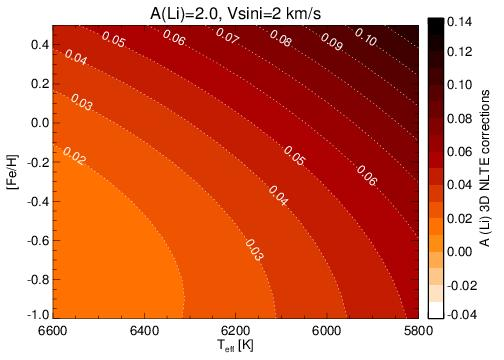# 3D non-LTE corrections for Li abundance and 6Li/7Li isotopic ratio in solar-type stars

### Tool explanation

This tool utilizes the analytical expressions developed in Harutyunyan G., Steffen M., Mott A. et al. (2018) to compute the 3D non-LTE (NLTE) corrections for lithium abundance (A(Li)) and 6Li/7Li isotopic ratio. The 3D NLTE correction of A(Li), defined as Δ A(Li), is computed as a function of effective temperature (Teff), metallicity ([Fe/H]) and 1D LTE A(Li), whereas the 3D NLTE correction of 6Li/7Li isotopic ratio, defined as Δ 6Li/7Li, is a function of Teff, [Fe/H], surface gravity (log g), projected rotational velocity (v sini), 1D LTE A(Li) and 6Li/7Li ratio.

The analytical expressions used by this tool were derived for a typical range of stellar parameters for solar-type stars, and they are valid for the following ranges of input parameters:

5800 ≤ Teff ≤ 6500 [K]

-1.0 ≤ [Fe/H] ≤ +0.5

4.0 ≤ log g ≤ 4.5

0 ≤ v sini ≤ 6 [km s -1]

1.0 ≤ A(Li) ≤ 2.7

0 ≤ 6Li/7Li ≤ 15 [%]

A(Li)3D NLTE = A(Li)1D LTE + Δ A(Li)

6Li/7Li3D NLTE = 6Li/7Li1D LTE + Δ 6Li/7LiContour plots of 3D NLTE A(Li) corrections, Δ A(Li) (upper panel), and 6Li/7Li ratio corrections, Δ 6Li/7Li (middle panel) in the Teff – [Fe/H] plane. The same 3D NLTE 6Li/7Li corrections in the Teff – log g plane are shown in the bottom panel. The contours are computed for [Fe/H]=0, v sini=2 km s-1, 1D LTE A(Li)=2.0 and 6Li/7Li=5%.